# SQL Server Mathematical functions (SQRT, PI, SQUARE, ROUND, CEILING & FLOOR)

Mathematical functions are present in SQL server which can be used to perform mathematical calculations. Some commonly used mathematical functions are given below:
1. SQRT():
SQRT() function is the most commonly used function. It takes any numeric values and returns the square root value of that number.

Syntax:

`SELECT SQRT(..value..)`

Example: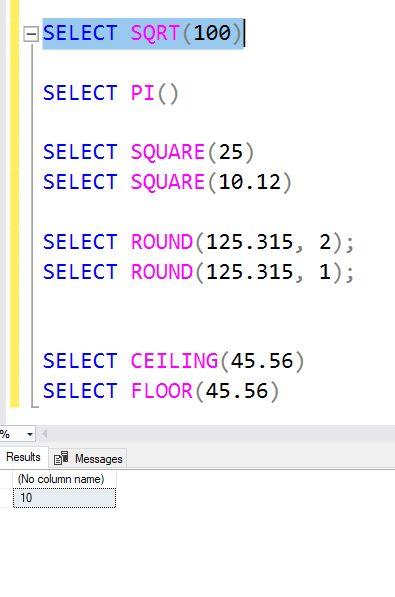2. PI(): There are calculations which require use of pi. Using pi() function, value of PI can be used anywhere in the query.

Syntax:

`SELECT PI()`

Example: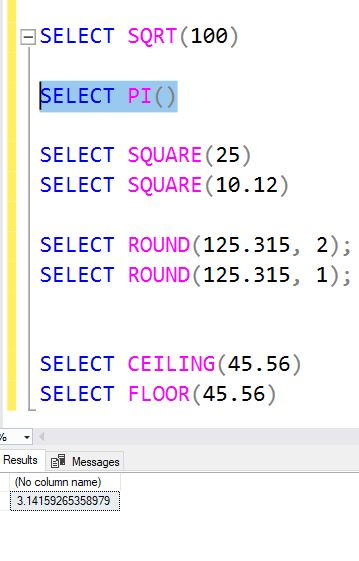3. SQUARE(): SQUARE() function is used to find the square of any number.

Syntax:

`SELECT SQUARE(..value..)`

Example: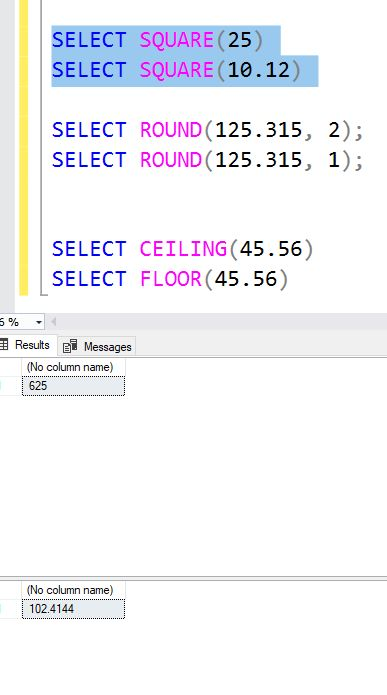4. ROUND(): ROUND() function is used to round a value to the nearest specified decimal place.

Syntax:

`SELECT ROUND(..value.., number_of_decimal_places)`

Example: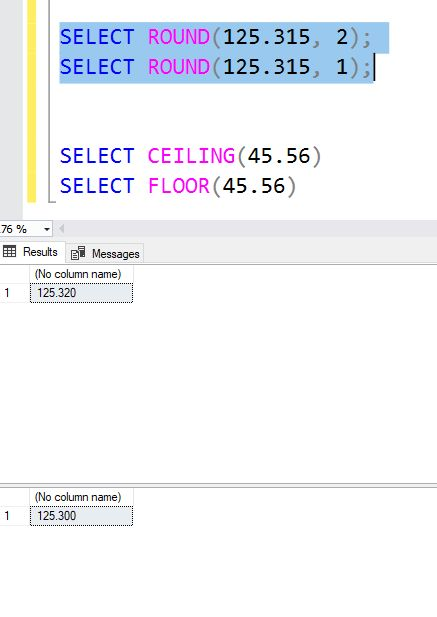5. CEILING() and FLOOR()
CEILING(): CEILING() function is used to find the next highest value (integer).

Syntax:

`SELECT CEILING(..value..)`

FLOOR(): FLOOR() function returns the next lowest value (integer).
Syntax:

`SELECT FLOOR(..value..)`

Example: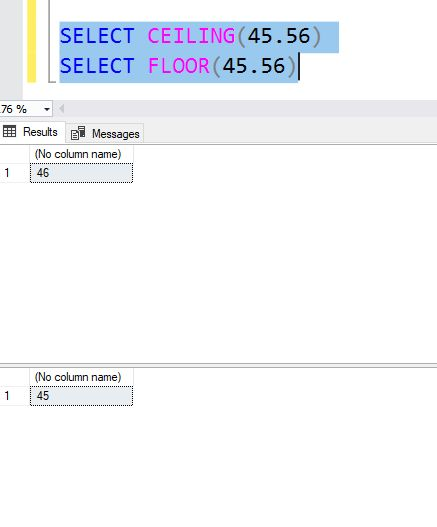My Personal Notes arrow_drop_upCheck out this Author's contributed articles.

If you like GeeksforGeeks and would like to contribute, you can also write an article using contribute.geeksforgeeks.org or mail your article to contribute@geeksforgeeks.org. See your article appearing on the GeeksforGeeks main page and help other Geeks.

Please Improve this article if you find anything incorrect by clicking on the "Improve Article" button below.

Article Tags :
Practice Tags :

2

Please write to us at contribute@geeksforgeeks.org to report any issue with the above content.